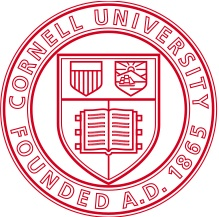# Rajit Manohar

### Puzzles:

Write all the integers from 0 to 100 using four 4's and mathematical symbols only. For instance, 0 = 4 + 4 - 4 - 4; 1 = 44/44; and so on. (Symbols: +, -, *, /, (, ), !, sqrt, ^, .)

48 is an interesting number. If you add 1, you get 49, a perfect square. If you divide it by 2 and then add 1, you get 25, another perfect square. What is the next integer with this property?

You are given 13 coins, 12 genuine and 1 counterfeit. It is given that the counterfeit coins and genuine coins have different weights. You are also given a scale which when given a pair (A,B) of disjoint subsets of the 13 coins returns how the weights of A and B compare (equal, greater, or less). If you are allowed at most 3 uses of the scale, how would you determine which coin is counterfeit? Can you generalize this problem? (With an appropriately generalized solution, of course!)

Find all the total functions f that map reals to reals that are not identically zero, and that satisfy the following relation: f((x+y)/(x-y)) = (f(x)+f(y))/(f(x)-f(y))

A chessboard can be tiled with 32 dominos. Suppose we remove the left top and right bottom corner of the board. Can the remaining 62 squares be tiled by 31 dominos?

You are given a bar of chocolate with 50 squares (5 x 10). What is the minimum number of breaks necessary to break the bar into 50 individual squares? A bar (or a piece of it) can only be broken along a straight line that runs from one side to the other. Pieces cannot be stacked while breaking.

Given two positive numbers, what is the probability that they are relatively prime? (Assume a uniform distribution.)

Let f be a total function from natural numbers to natural numbers. It has the property that f(f(n)) < f(n+1) for all n. Find a simple characterization of f.

Show that a path on an m by n square grid which starts at the northwest corner, goes through each point exactly once, and ends at the southeast corner divides the grid into two equal halves: (a) those regions opening north and east; and (b) those regions opening south or west.

Adam, Rajit, Rob and Rohit are sitting on the beach at Malibu around 3am.
Rob:
"I just picked two integers greater than one."
"Rohit, their product is..." (he whispers it to Rohit).
"Rohit, we don't know the numbers."
Rohit:
"Now I do."